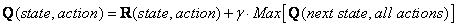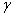## What is Q-Learning

In the previous sections of this tutorial, we have modeled the environment and the reward system for our agent. This section will describe learning algorithm called Q learning (which is a simplification of reinforcement learning).

We have model the environment reward system as matrix R.Now we need to put similar matrix name Q in the brain of our agent that will represent the memory of what the agent have learned through many experiences. The row of matrix Q represents current state of the agent, the column of matrix Q pointing to the action to go to the next state.

In the beginning, we say that the agent know nothing, thus we put Q as zero matrix. In this example, for the simplicity of explanation, we assume the number of state is known (to be six). In more general case, you can start with zero matrix of single cell. It is a simple task to add more column and rows in Q matrix if a new state is found.

The transition rule of this Q learning is a very simple formulaThe formula above have meaning that the entry value in matrix Q (that is row represent state and column represent action) is equal to corresponding entry of matrix R added by a multiplication of a learning parameterand maximum value of Q for all action in the next state.

Tired of ads? Read it off line on any device. Click here to purchase the complete E-book of this tutorial

Preferable reference for this tutorial is

Teknomo, Kardi. 2005. Q-Learning by Examples. http://people.revoledu.com/kardi/tutorial/ReinforcementLearning/index.html